# Operationalization

## Site characteristics

With ‘site characteristics’, characterising aspects are meant of airports. The airport characteristics ‘capacity’ and ‘facilities’ can not be operationalized because the correct data is unavailable. The number of facilities and capacity do relate to the airport hierarchy. For example, primary airports offer more facilities compared to tertiary airports. The other characteristics can be operationalized and will be discussed in this section. These characteristics are (click on a link to jump to the related subject):

• Turn-around time
• Proportion
• Distance and frequency
• Competition
• Hierarchy
• ### Turn-around time

Although there is no exact data of low-cost carrier turn-around times at airports, these can be estimated to a certain degree. In this research the turn-around time of a low-cost carrier will be roughly estimated by calculating the average flight frequency for each 500 kilometres and for each 60 minutes: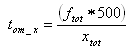and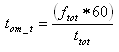where: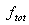= total number of low-cost carrier flights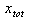= total distance in kilometres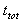= total travel time in minutes

The turn-around time for each 500 kilometres will be calculated by multiplying the total frequency with 500 and divide it with the total distance. The higher the value, the shorter the average turn-around time. In case of the turn-around time for each 60 minutes the total frequency needs to be multiplied with 60 and divided with the total travel time.

### Proportion

At each airport will be looked at the proportion of the total amount of seats of incoming and departing low-cost carrier flights. Next this will be related to the total amount of seats of other carriers. This way a percentage of the number of seats of low-cost carriers related to other carriers can be given of each airport. These densities can be calculated according: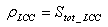and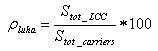where: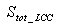= total number of low-cost carrier seats at a given airport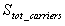= total number of carrier seats at a given airport

Another measure is the theta index: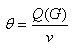This measure is an indication of the amount of traffic at an airport. A high value indicates heavy traffic on the network. More information on the theta index can be found here.

### Distance and frequency

The distance and frequency at airports will be analysed on a similar way as is the case with the ‘links’. This way the results can be compared and checked if there are differences.

### Hierarchy

By dividing airports into primary, secondary, and tertiary an airport hierarchy can be created. This division will be based on the total number of seats of all carriers on an airport. An alternative method would be to use a similar hierarchy based on only low-cost carriers. The airport hierarchy is based on a hierarchy used by SEO Economical Research.

### Competition

For each airport the amount of competing low-cost carrier flights can be analysed. The market share of low-cost carriers at an airport can be calculated with the Herfindahl index 8. This index is an indication of competition between carriers at different airports: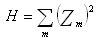where:

z = the market share of a carrier (calculated by dividing the number of available seats of a carrier with the total number of seats of all carriers)
m = the number of carriers

The resulting value of the index will be between 0 and 1. A value close to 0 would indicate a high potential of competition. A value close to 1 would indicate almost no competition.

### -Top-

8In this research the herfindahl index is calculated with help of Wessa (2006).